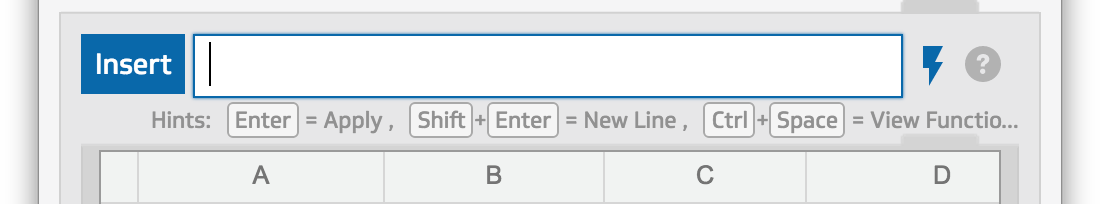# Introduction to the formula bar

The formula bar is used in the Klip Editor (to build Klips) and in the modeller (to model data sources for  custom metrics and Klips). In the formula bar you build formulas, using functions, to manipulate, refine, and perform calculations on your data. See How to use the formula bar for more detailed information.

Note: For the most part, the examples in this article refer to using the formula bar within the Klip Editor, however, the same features are applicable when writing formulas in the modeller.## How do I build and edit formulas?

In the formula bar, you can type-in formulas or select from a list of functions. When you start typing a function name in the formula bar, a list of functions filtered by the letter you entered is displayed. Online help with examples and parameters automatically display for each function in a pop-up window.

To start building a formula, you can click on Insert or just start typing in the formula bar. When you select Function from the Insert menu, a list of functions displays. The following example show you how to start building a formula in the Klip Editor:If you know the function you want, just start typing.## How do I view all the available functions?

To see all the functions available for building formulas, click Insert, select Functions and then click on the Browse All Functions link. The Function Browser opens in the right-hand sidebar.